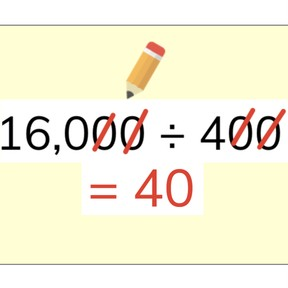Dividing numbers that are multiples of 10

# Dividing numbers that are multiples of 10

I can divide multi-digit whole numbers that end with zeros.8,000 schools use Gynzy92,000 teachers use Gynzy1,600,000 students use Gynzy

## General

In this lesson, students will identify the basic fact of an equation and use their knowledge of place value to complete the division of numbers that are multiples of 10. They will learn the strategy to cancel out zeros when dividing multiples of 10.

## Standards

CCSS.MATH.CONTENT.4.NBT.A.1

## Learning Objective

Students will be able to use mental math strategies to divide multi-digit numbers such as 80 divided by 2 and 350 divided by 70.

## Introduction

The lesson begins with a review of multiples of 10. Students highlight to identify all the multiples of 10 on the board. Next, review some division vocabulary: dividend, divisor, and quotient. Then explain inverse operations and discuss how using multiplication can help students easily learn basic division facts. Finally, students review basic division facts and try to solve basic facts while beating the 2-minute timer.

## Instruction

To divide numbers that have dividends that end in zero, follow 3 steps. First, find the basic fact and write the quotient. Next, count the zeros in the dividend, and finally, write the zeros after the basic fact’s quotient. Students are presented with an example and told that they can check their work with inverse operations. After this, warn students to watch out for basic facts that contain zeros. The zeros in the basic facts are not part of the quotient. Students participate in interactive activities to check their understanding. Next, students practice with divisors that end with zeros by crossing out the zeros evenly on either side of the ÷ sign, starting in the one’s place. Then count the zeros that are left. Students practice striking out the zeros and solving equations.

## Quiz

Students respond to ten multiple-choice questions as well as fill-in responses in which students solve division problems.

## Closing

The lesson concludes with a fun math riddle. Students drag letters to the correct answers to each equation. The letters will spell out the answer to the riddle, “Which month has 28 days?” Check the following slide to see the answer!

### The online teaching platform for interactive whiteboards and displays in schools

• Save time building lessons

• Manage the classroom more efficiently

• Increase student engagement